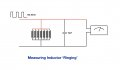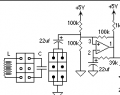# Finding the resonant frequency of some coils

#### JulesP

Joined Dec 7, 2018
374
Hi there,

I have been exploring the properties of the HV Back EMF pulses created by coils fed with a square wave input using a circuit I have constructed and which has benefited from some great advice from this forum (Battery swapper and coil driver amplifier).

In my measurements of the energy released into a battery by the Back EMF pulses, I am trying to find the resonant frequency of the coils so I can narrow my focus of the input frequency and wondered if there was a straight forward way to find this frequency practically. Bearing in mind that I am using a pulsed DC input (square wave of typically 50% duty cycle) and not a sine wave, I'm hoping there is a simple way to do it.

I will describe what I have in the circuit to clarify. The coils are each made up of about 500 turns of 0.71mm wire wound on ferrite cores and built up in five layers (see pics); the white paper makes it easier to see and wind them cleanly. The 7 coils each have a resistance of just under 2 Ohms and they are connected in parallel and as such have a combined resistance of about 0.5 ohms. They are fed with 12V DC from one or other of the batteries and the FET gate is driven by the square wave generator at between 20-60% duty cycle. When the FET is triggered the coils all conduct and when the FET switches off the resulting combined Back EMF pulse is directed through some diodes to one or other of the batteries. The battery swapper makes sure that the battery providing the energy for the circuit is not the one receiving the HV pulses and they switch over every 2 mins. I recognise that there is a 'flyback' diode in the FET that seeks to limit these BEMF pulses but even so the HV output is over 550V (see waveform pic - using a 20:1 potential divider) and of small pulse width producing a high voltage gradient (the effect of these high gradients is what I'm investigating).

The actual circuit pic shows my original construction with 7 FET channels which were cycled round using a CD4017 chip as I reasoned that the current through the coils in parallel would be very high (12/0.5=24A). In practice, even with all 7 in parallel, the circuit current at 200Hz is less than 0.5A so I am just using one FET channel now. At higher frequencies the current drops even further.

My query is how to find the resonant frequency of the seven coils together so I can zoom in on that input frequency range. If I knew the overall inductance and capacitance I could calculate the theoretical value but it might be easier to measure it directly in some way. I could I suppose measuring the HV pulses on the scope, as I have done, and increment the input frequency 5Hz at a time to find where the pulses are highest but that could take a long time.

Any thoughts would be appreciated.

Jules

Last edited:

#### wayneh

Joined Sep 9, 2010
17,153
I built a device a while back called a ring tester. It puts a capacitor across the poles of an inductor, hits it with a brief pulse, and then counts the ringing oscillations before they fade below a threshold or before the timing window ends. It's thus limited to testing within a certain range of inductance since a large inductor would give big, slow oscillations and only ring a time or two before the end of the timing window.

Anyway, it seems like you could estimate the inductance by a similar process. Put a known capacitance across them, ping them, and look at the period of the oscillations as they ring.

#### JulesP

Joined Dec 7, 2018
374
Sounds interesting. I think you are saying that if I place a known capacitance across the ends of my parallel coils and send some sort of pulse in then the resulting 'ringing' frequency will allow me to calculate backwards what the inductance is and from that then calculate the resonant frequency.

Would I use my present signal generator to provide the pulse and connect a scope across the ends of the coils to see the 'ringing' (as per diagram)?#### Alec_t

Joined Sep 17, 2013
12,148
My query is how to find the resonant frequency of the seven coils together
Are you interested in the self-resonant frequency of the coil assembly alone, or the resonant frequency of the whole caboodle ... coils plus diodes plus battery-swapper? I would expect the two frequencies to be quite different, if the relatively small self-capacitance of the coils is swamped by the external capacitance. I'm not sure how useful knowing the self-resonant frequency would be, given that the coils in use will be loaded by the rest of the circuit.

#### DickCappels

Joined Aug 21, 2008
7,538
Sounds interesting. I think you are saying that if I place a known capacitance across the ends of my parallel coils and send some sort of pulse in then the resulting 'ringing' frequency will allow me to calculate backwards what the inductance is and from that then calculate the resonant frequency.

Would I use my present signal generator to provide the pulse and connect a scope across the ends of the coils to see the 'ringing' (as per diagram)?

View attachment 177718
It doesn't quite work that way. The method you (more properly JulesP) describe will allow you to find the inductance but not the resonant frequency.

If you ping the coil with narrow pulses through a sizeable resistance, or better yet a current source, you should see ringing, and that will be very close to the natural self-resonant frequency.

I've also done this by manually sweeping the signal generator while watching for the peak response which will be at the resonant frequency.

The resistor and your scope probe will affect the resonant frequency, and the resonant frequency is probably different for small ringing signals than for high current pulses because the permeability of the core is a function of ampere-turns.Another, related means of finding the self resonant frequency places the inductor in an oscillator and continually pings it at the resonant frequency. Use the circuit above with a comparator such as the LM393 and measure the frequency on the output.

Last edited:

#### JulesP

Joined Dec 7, 2018
374
Are you interested in the self-resonant frequency of the coil assembly alone, or the resonant frequency of the whole caboodle ... coils plus diodes plus battery-swapper? I would expect the two frequencies to be quite different, if the relatively small self-capacitance of the coils is swamped by the external capacitance. I'm not sure how useful knowing the self-resonant frequency would be, given that the coils in use will be loaded by the rest of the circuit.
Hi Alec, that's an interesting question. I have assumed that I need to find the resonant frequency of the coil assembly alone but in fact it may be very relevant to know both to experiment.

#### JulesP

Joined Dec 7, 2018
374
It doesn't quite work that way. The method you (more properly JulesP) describe will allow you to find the inductance but not the resonant frequency.

If you ping the coil with narrow pulses through a sizeable resistance, or better yet a current source, you should see ringing, and that will be very close to the natural self-resonant frequency.

I've also done this by manually sweeping the signal generator while watching for the peak response which will be at the resonant frequency.

The resistor and your scope probe will affect the resonant frequency, and the resonant frequency is probably different for small ringing signals than for high current pulses because the permeability of the core is a function of ampere-turns.

View attachment 177730
Another, related means of finding the self resonant frequency places the inductor in an oscillator and continually pings it at the resonant frequency. Use the circuit above with a comparator such as the LM393 and measure the frequency on the output.
From what you say it seems it might be just as easy to just ramp up the input signal 1 Hz at a time until I get the best HV Back EMF pulses?

#### DickCappels

Joined Aug 21, 2008
7,538
1 Hz is probably way too fine granularity to be practical, but yes,something along those lines.

We don't know exactly what you want to find out, so if you can add any detail to your request you can probably get more detail in the replies.

#### JulesP

Joined Dec 7, 2018
374
1 Hz is probably way too fine granularity to be practical, but yes,something along those lines.

We don't know exactly what you want to find out, so if you can add any detail to your request you can probably get more detail in the replies.
The waveform I showed in the original post showed a peak voltage of 28.4V (over 550 in actuality with a 20:1 divider) with an input signal of 199Hz so I am expecting a significantly higher peak voltage at resonance. If I go up in increments of 10Hz maybe I will find it but I had hoped for an easy way to identify the approximate resonant frequency.

Jules

#### DickCappels

Joined Aug 21, 2008
7,538
Build the circuit in post #5 and read the frequency directly.

#### MrAl

Joined Jun 17, 2014
8,353
Hello,

The ringing frequency will be different than the actual resonant frequency.

For the circuit in post #3 we have:

The resonant frequency is w=1/sqrt(L*C)

and the ringing frequency is w=sqrt(L*(4*C*R^2-L))/(2*C*L*R)

and so L in the resonant case is calculated from L=1/(w^2*C)

and L in the ringing case is calculated from L=(4*C*R^2)/(4*w^2*C^2*R^2+1)

So depending on what we measure we use a different formula.

If you want to read the ringing frequency and use it as the resonant frequency and get within a certain percentage pc, then you have to ensure that R is equal to or greater than:
sqrt(L)/(2*sqrt(1-a^2)*sqrt(C))

where
a is 1-pc

where pc is fractional percentage like 0.05 for 5 percent.
So if you want to get within 5 percent you make a=0.95, and if within 1 percent a=0.99, etc.
If R is made in that fashion you get a reasonable result, or else just use the more complicated formula.
In any case, there will be errors in the measurements of C and other variables anyway and also parasitics not included.

#### Wolframore

Joined Jan 21, 2019
2,317
feed it white noise and watch for areas that have higher output (amplification) on a spectrum analyzer.